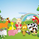1093瀏覽
Introduction

Calculating distances in signal processing/statistics/time-series analysis imply measuring the distance between two probability distribution, i am not really familiar with distances but since some formulas are in closed form they can be easily used for volatility estimation. This volatility indicator will use three methods originally made to measure the distance of gaussian copulas, using those methods for volatility estimation is fairly easy and provide a different approach to statistical dispersion.

The indicator have a length parameter and a method parameter to select the method used for volatility estimation, i describe each methods below.

Hellinger Method

Each method will use the rolling sum of the low price and the rolling sum of the high price instead of probability distributions. The Hellinger method have many application from the measurement of distances to the use as a cost function for neural networks.

Its closed form is defined as the square root of 1 - a^0.25b^0.25/(0.5a + 0.5b)^0.5 where a and b are both positive series. In our indicator a is the rolling sum of the high price and b the rolling sum of the low price. This method give a classic estimation of volatility .

Bhattacharyya Method

The Bhattacharyya method is another method who use a natural logarithm, this method can visually filter small volatility variation. It is defined as 0.5 * log((0.5a+0.5b)/√(ab)).

Wasserstein Method

This method was originally using a trimmed mean for its calculation. The original method is defined as the square of the trimmed mean of a + b - 2√(a^0.5ba^0.5), a median has been used instead of a trimmed mean for efficiency sake, both central tendency estimators are robust to outliers.

Conclusion

I showed that closed form formulas for distance calculation could be derived into volatility estimators with different properties. They could be used with series in a range of (0,1) to provide a smoothing variable for exponential smoothing.

Patreon: https://www.patreon.com/alexgrover